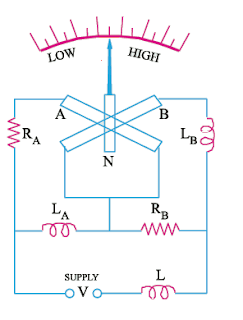# Analog Frequency Meter Working Principle

Hi friends, in this article, I am discussing about analog frequency meter working principle and hope you will find it informative and helpful.

The action of moving iron analog frequency meter depends on the variation in current drawn by two parallel circuits – one inductive and the other non-inductive – when the frequency changes.

# Construction of moving iron analog frequency meterThe construction and internal connections are shown in Figure. The two coils A and B are so fitted that their magnetic axes are perpendicular to each other. At their centers, a long and thin soft-iron pointer is pivoted, which aligns itself along the resultant magnetic field of the two coils. No controlling torque is provided in this instrument.

It will be noted that the various circuit elements constitute a Wheatstone bridge which becomes balanced at the supply frequency. Coil A has a resistance RA in series with it and a coil LA in parallel. Similarly, RB is in series with coil B and LB is in parallel.

The series inductance L helps to suppress higher harmonics in the current waveform and hence, tends to minimize the waveform errors in the indication of the instrument.

# Analog frequency meter working principle

When the instrument is connected to the supply, currents pass through coils A and B and produce opposing torques. When supply frequency is high, currents through coil A is more whereas that through coil B is less due to the increase in the reactance offered by LB.

Hence, the magnetic field of coil A is stronger than that of coil B. Consequently, the iron needle lies more nearly to the magnetic axis of coil A than that of B.

For low frequencies, coil B draws more current than coil A and, hence, the needle lies more nearly parallel to the magnetic axis of B than to that of coil A.

The variations of frequency are followed by the pointer as explained above.

The instrument can be designed to indicate a broad or narrow range of frequencies determined by the parameters of the circuit.